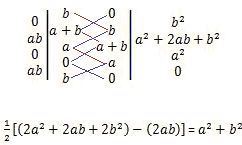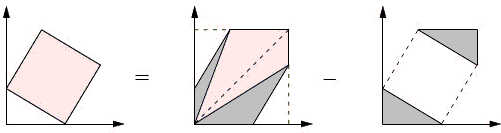Pythagorean Theorem from the Shoelace Formula

John Molokach also observed that the Pythagorean theorem follows from Gauss' Shoelace Formula. The formula gives the area of a polygon A1A2...An in the Cartesian plane, where vertices are defined by their coordinates, Aj = (xj, yj), j = 1, ..., n:

Area(A1A2...An) = ½|∑(xjyj+1 - xj+1yj)| = ½|∑xj(yj+1 - yj-1)|,

where the sum is taken for j = 1, ..., n and the indices are defined cyclically: xn+1 = x1 and x0 = xn and similarly for y's.

For the square with vertices A1(b, 0), A2(a+b, b), A3(a, a+b), A4(0, a), the formula givesOn the other hand, if c is the hypotenuse of the right triangle with vertices (0, 0), (b, 0), and (0, a) then the area of the square formed on the hypotenuse is c², showing that indeed a² + b² = c².

A question could be asked whether the Shoelace formula, in itself, is based on the Pythagorean theorem, causing a vicious circle in proving the latter. Eschewing this question for the time being, we present Roger Nelsen's synthetic proof without words that algebraically is equivalent to the above derivation:In the first figure on the right, one of the darker triangles has area a²/2, the other one - b²/2. The light triangles have areas a(a + b)/2 and b(a + b)/2, giving together (a + b)²/2. Each of the triangles in the right figure has area of ab/2. This we get, for the area of the square c×c on the left

a²/2 + b²/2 + (a + b)²/2 - 2ab/2 = a² + b².